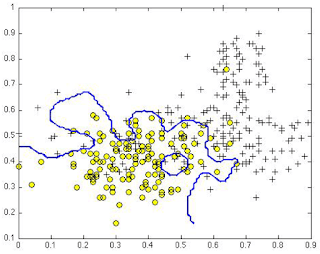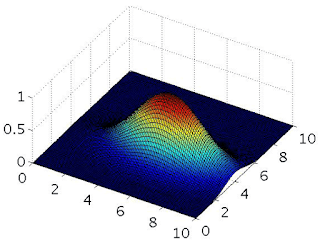# ▸ Support Vector Machines :

1. Suppose you have trained an SVM classifier with a Gaussian kernel, and it learned the following decision boundary on the training set:You suspect that the SVM is underfitting your dataset. Should you try increasing or decreasing ? Increasing or decreasing ?

• It would be reasonable to try decreasing C. It would also be reasonable to try increasing .

• It would be reasonable to try decreasing C. It would also be reasonable to try decreasing .

• It would be reasonable to try increasing C. It would also be reasonable to try decreasing .
The figure shows a decision boundary that is underfit to the training set, so we’d like to lower the bias / increase the variance of the SVM. We can do so by either increasing the parameter C or decreasing .

• It would be reasonable to try increasing C. It would also be reasonable to try increasing .

1. Suppose you have trained an SVM classifier with a Gaussian kernel, and it learned the following decision boundary on the training set:When you measure the SVM’s performance on a cross validation set, it does poorly. Should you try increasing or decreasing ? Increasing or decreasing ?

• It would be reasonable to try decreasing C. It would also be reasonable to try increasing .
The figure shows a decision boundary that is overfit to the training set, so we’d like to increase the bias / lower the variance of the SVM. We can do so by either decreasing the parameter C or increasing .

• It would be reasonable to try decreasing C. It would also be reasonable to try decreasing .

• It would be reasonable to try increasing C. It would also be reasonable to try decreasing .

• It would be reasonable to try increasing C. It would also be reasonable to try increasing .

1. The formula for the Gaussian kernel is given by similarity
.
The figure below shows a plot of when .Which of the following is a plot of when ?

• Figure 1:• Figure 2:This figure shows a “narrower” Gaussian kernel centered at the same location which is the effect of decreasing .

• Figure 3:• Figure 4:1. The SVM solves

where the functions and look like this:The first term in the objective is:

This first term will be zero if two of the following four conditions hold true. Which are the two conditions that would guarantee that this term equals zero?

• For every example with = 0, we have that θT ≤ −1.
For examples with = 0, only the term is present. As you can see in the graph, this will be zero for all inputs less than or equal to -1.

• For every example with = 1, we have that θT ≥ 0.

• For every example with = 0, we have that θT ≤ 0.

• For every example with = 1, we have that θT ≥ 1.
For examples with = 1, only the term is present. As you can see in the graph, this will be zero for all inputs greater than or equal to 1.

1. Suppose you have a dataset with n = 10 features and m = 5000 examples.

After training your logistic regression classifier with gradient descent, you find that it has underfit the training set and does not achieve the desired performance on the training or cross validation sets.

Which of the following might be promising steps to take? Check all that apply.

• Increase the regularization parameter λ.

• Use an SVM with a Gaussian Kernel.
By using a Gaussian kernel, your model will have greater complexity and can avoid underfitting the data.

• Create / add new polynomial features.
When you add more features, you increase the variance of your model, reducing the chances of underfitting.

• Use an SVM with a linear kernel, without introducing new features.

• Try using a neural network with a large number of hidden units.
A neural network with many hidden units is a more complex (higher variance) model than logistic regression, so it is less likely to underfit the data.

• Reduce the number of example in the training set.

### Check-out our free tutorials on IOT (Internet of Things):

1. Which of the following statements are true? Check all that apply.

• Suppose you are using SVMs to do multi-class classification and would like to use the one-vs-all approach. If you have K different classes, you will train K-1 different SVMs.

• If the data are linearly separable, an SVM using a linear kernel will return the same parameters θ regardless of the chosen value of C (i.e., the resulting value θ of does not depend on C).

• It is important to perform feature normalization before using the Gaussian kernel.
The similarity measure used by the Gaussian kernel expects that the data lie in approximately the same range.

• The maximum value of the Gaussian kernel (i.e., ) is 1.
When , the Gaussian kernel has value , and it is less than 1 otherwise.

• Suppose you have 2D input examples (ie, ). The decision boundary of the SVM (with the linear kernel) is a straight line.
The SVM without any kernel (ie, the linear kernel) predicts output based only on , so it gives a linear / straight-line decision boundary, just as logistic regression does.

• If you are training multi-class SVMs with one-vs-all method, it is not possible to use a kernel.

&
Click here to see more codes for Raspberry Pi 3 and similar Family.
&
Click here to see more codes for NodeMCU ESP8266 and similar Family.
&
Click here to see more codes for Arduino Mega (ATMega 2560) and similar Family.

Feel free to ask doubts in the comment section. I will try my best to answer it.
If you find this helpful by any mean like, comment and share the post.
This is the simplest way to encourage me to keep doing such work.

Thanks & Regards,
- APDaga DumpBox

1.1.2.